11-3 LDA (嚙踝蕭嚙踝蕭嚙踝蕭嚙踝蕭嚙踝蕭嚙踝蕭嚙踝蕭嚙踝蕭嚙踝蕭)

chinese version

If we treat each data entry as a point in high-dimensional space, then we shall be able to visualize the dataset if the dimensionality is less than or equal to 3. For instance, the following example plots the dataset (3 dimensions, 300 entries) with 3 class labels using different viewing angles:

Example 1: inputExtraction01.mDS=prData('random3'); subplot(1,2,1); dsScatterPlot3(DS); view(155, 46); subplot(1,2,2); dsScatterPlot3(DS); view(-44, 22);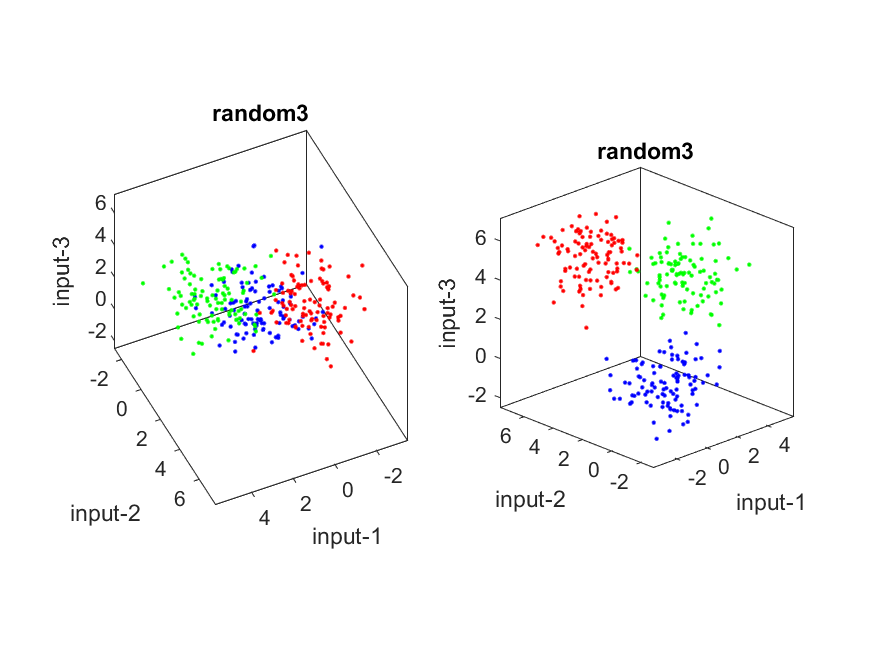The left and right plots are based on the same 300 entries except for different view angles. The left plot seems a bit random since the view angle tends to overlap the projection onto the 2D space. On the otherhand, the right plot give us a much more separation between classes after 2D projection. As you can imagine, the goal of LDA is to find the best projection (or equivalently, the best viewing angle) such that the separation between classes is maximized.

Hint
In the above example, you can try to click and drag the plot axis to change the viewing angle. Try it and see which viewing angle can give you the best separation between data of different classes.

The ultimate goal of feature extraction can be explained in a mathematical manner. Suppose that we have a dataset with features of V = {v1 , v2 ,... , vd}. We wish to have a classifier with recognition rate denoted by J(˙), which is a function of the transform features. Therefore the objective of feature extraction is to find the best of transformed features S such that J(S)≧J(T), where both S and T are sets of linearly transformed features obtained from set V.

Let us use LDA to project the dataset used in the previous example to 2D space:

Example 2: ldaRandom301.mDS=prData('random3'); DS2=lda(DS); DS2.input=DS2.input(1:2, :); DS3=lda(DS); DS3.input=DS3.input(end-1:end, :); subplot(2,1,1); dsScatterPlot(DS2); axis image xlabel('Input 1'); ylabel('Input 2'); title('Projected to the first two dim of LDA'); subplot(2,1,2); dsScatterPlot(DS3); axis image xlabel('Input 3'); ylabel('Input 4'); title('Projected to the last two dim of LDA');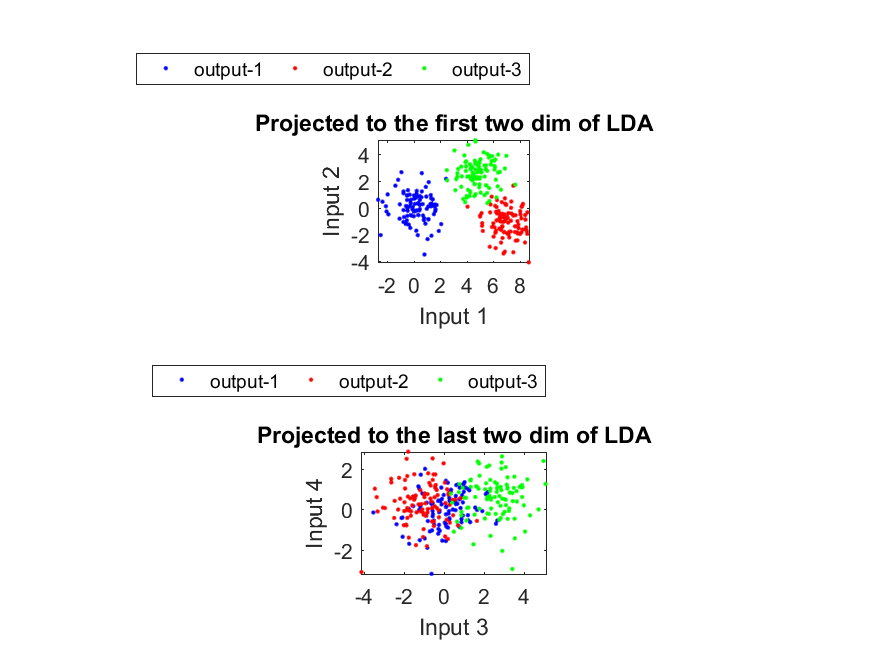When we do LDA projection to the first dimensions, it is obvious that the separation between different classes in plot 1 is much larger than that in plot 2.

The next example applies LDA to IRIS dataset:

Example 3: ldaIris2d01.mDS = prData('iris'); dataNum = size(DS.input, 2); DS2 = lda(DS); % ====== Projection to the first two eigenvectors DS3=DS2; DS3.input=DS2.input(1:2, :); subplot(2,1,1); [recogRate, computed] = knncLoo(DS3, [], 1); title(sprintf('LDA projection of %s data onto the first 2 discriminant vectors', DS.dataName)); xlabel(sprintf('KNNC''s leave-one-out recog. rate = %d/%d = %g%%', sum(DS3.output==computed), dataNum, 100*recogRate)); % ====== Projection to the last two eigenvectors DS3=DS2; DS3.input=DS2.input(end-1:end, :); subplot(2,1,2); [recogRate, computed] = knncLoo(DS3, [], 1); title(sprintf('LDA projection of %s data onto the last 2 discriminant vectors', DS.dataName)); xlabel(sprintf('KNNC''s leave-one-out recog. rate = %d/%d = %g%%', sum(DS3.output==computed), dataNum, 100*recogRate));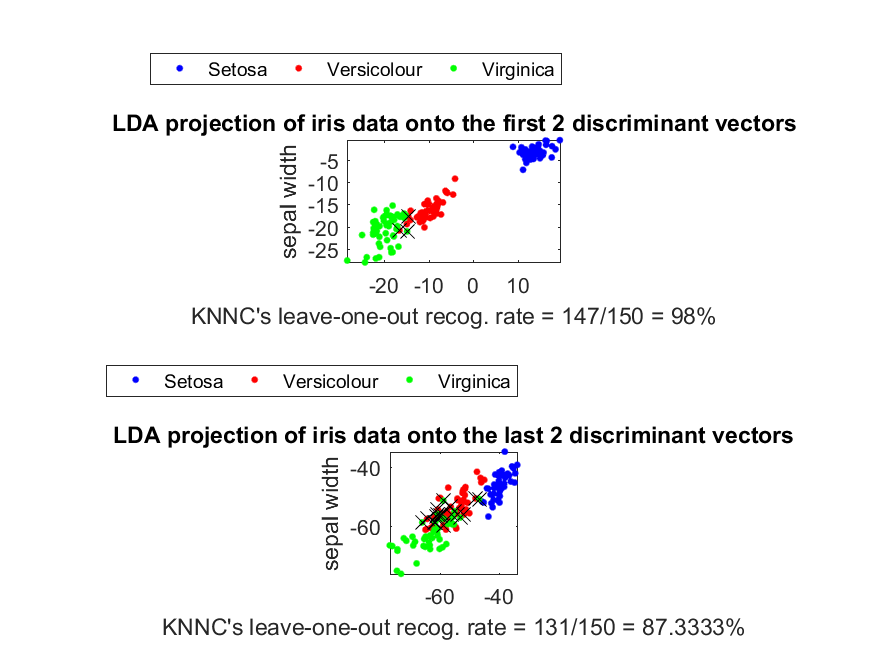In the above example, we adopt 1-nearest-neighbor classifier and leave-one-out criterion for performance evaluation. In plot 1, the dataset is projected to the first 2 dimensions of LDA and the accuracy is 98.00%, corresponding to 3 misclassified cases. If the dataset is projected to the last 2 dimensions of LDA, as shown in plot 2 where the degree of class overlap becomes larger, the accuracy is 87.33%, corresponding to 19 misclassified cases. (In the plot, the misclassified cases are denoted by a black cross.)

We can apply the same procedure for analyzing WINE dataset using LDA:

Example 4: ldaWine2d01.mDS = prData('wine'); dataNum = size(DS.input, 2); DS2 = lda(DS); % ====== Projection to the first two eigenvectors DS3=DS2; DS3.input=DS2.input(1:2, :); subplot(2,1,1); [recogRate, computed] = knncLoo(DS3, [], 1); title(sprintf('LDA projection of %s data onto the first 2 discriminant vectors', DS.dataName)); xlabel(sprintf('KNNC''s leave-one-out recog. rate = %d/%d = %g%%', sum(DS3.output==computed), dataNum, 100*recogRate)); % ====== Projection to the last two eigenvectors DS3=DS2; DS3.input=DS2.input(end-1:end, :); subplot(2,1,2); [recogRate, computed] = knncLoo(DS3, [], 1); title(sprintf('LDA projection of %s data onto the last 2 discriminant vectors', DS.dataName)); xlabel(sprintf('KNNC''s leave-one-out recog. rate = %d/%d = %g%%', sum(DS3.output==computed), dataNum, 100*recogRate));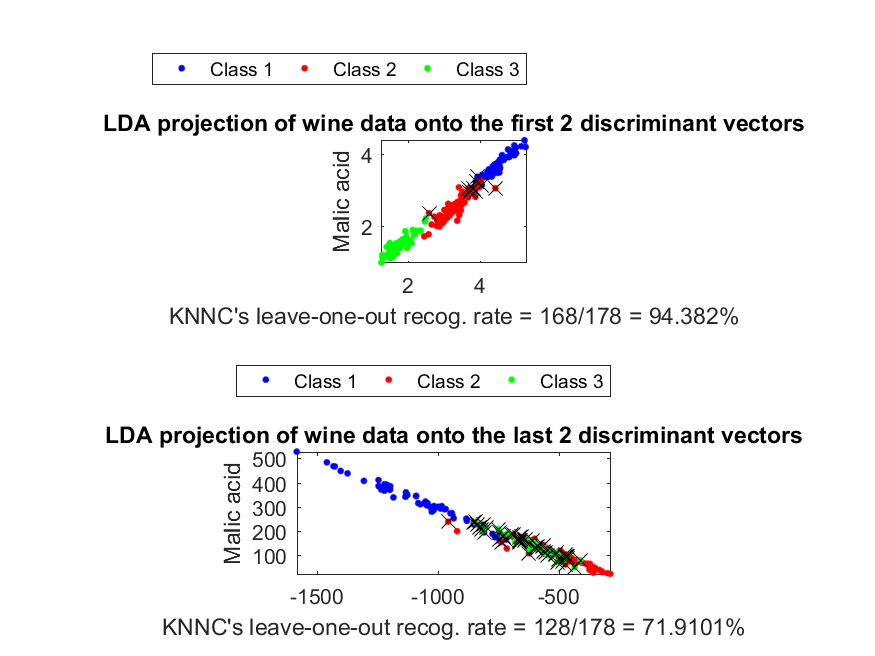Again, the projection along the first 2 dimensions has better class separation.

The following example demonstrate the effects of LDA dimension reduction with respect to classification accuracy (obtained via KNNC and leave-one-out criterion) of IRIS dataset:

Example 5: ldaIrisDim01.mDS=prData('iris'); [featureNum, dataNum] = size(DS.input); [recogRate, computed] = knncLoo(DS); fprintf('All data ===> LOO recog. rate = %d/%d = %g%%\n', sum(DS.output==computed), dataNum, 100*recogRate); DS2 = lda(DS); recogRate=[]; for i = 1:featureNum DS3=DS2; DS3.input=DS3.input(1:i, :); [recogRate(i), computed] = knncLoo(DS3); fprintf('LDA dim = %d ===> LOO recog. rate = %d/%d = %g%%\n', i, sum(DS3.output==computed), dataNum, 100*recogRate(i)); end plot(1:featureNum, 100*recogRate, 'o-'); grid on xlabel('No. of projected features based on LDA'); ylabel('LOO recognition rates using KNNC (%)');All data ===> LOO recog. rate = 144/150 = 96% LDA dim = 1 ===> LOO recog. rate = 143/150 = 95.3333% LDA dim = 2 ===> LOO recog. rate = 147/150 = 98% LDA dim = 3 ===> LOO recog. rate = 142/150 = 94.6667% LDA dim = 4 ===> LOO recog. rate = 144/150 = 96%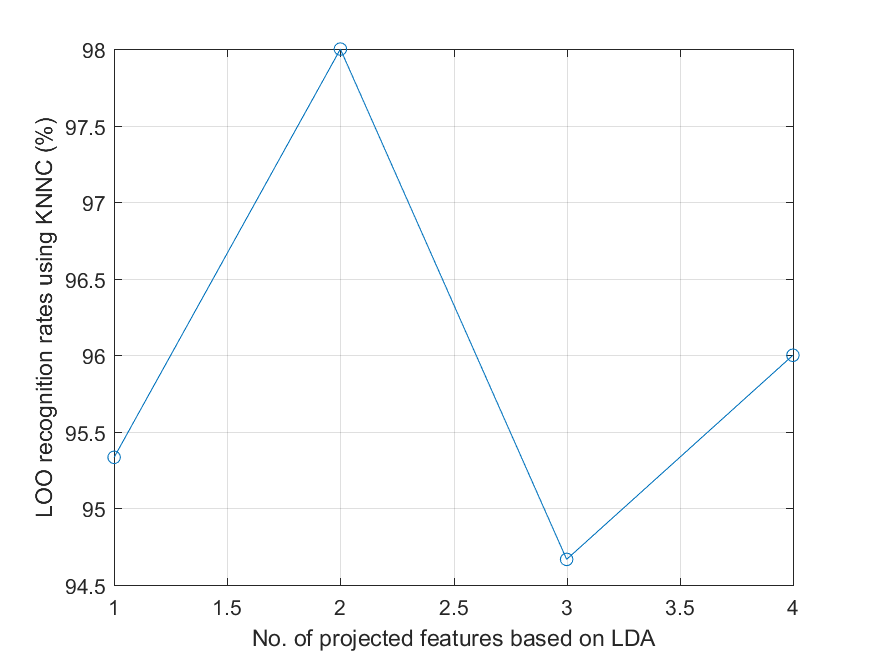From the plot, we know that the accuracy achieves its maximum at 98.00% when the dimensionality is 2. The corresponding confusion matrix is obtained as follows:

Example 6: ldaIrisConf01.mDS=prData('iris'); DS2 = lda(DS); DS3=DS2; DS3.input=DS3.input(1:2, :); [recogRate, computedOutput] = knncLoo(DS3); confMat=confMatGet(DS3.output, computedOutput); opt=confMatPlot('defaultOpt'); opt.className=DS.outputName; confMatPlot(confMat, opt);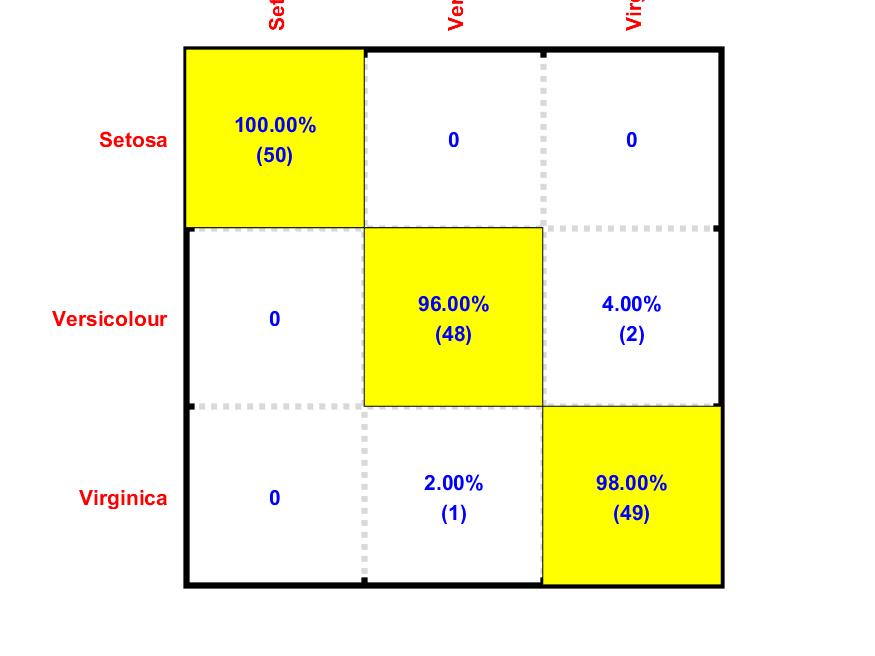If we denote matrices in plot 1 and 2 as A and B, respectively, then A(i,j) is the count of class i being classified as class j, while B(i, j) is the probability of class i being classified as class j, satisfying B(i, 1) + B(i, 2) + B(i, 3) = 100% for all i。

If we apply the same procedure to WINE dataset, the results are shown next:

Example 7: ldaWineDim01.mDS=prData('wine'); [featureNum, dataNum] = size(DS.input); [recogRate, computed] = knncLoo(DS); fprintf('All data ===> LOO recog. rate = %d/%d = %g%%\n', sum(DS.output==computed), dataNum, 100*recogRate); DS2 = lda(DS); recogRate=[]; for i = 1:featureNum DS3=DS2; DS3.input=DS3.input(1:i, :); [recogRate(i), computed] = knncLoo(DS3); fprintf('LDA dim = %d ===> LOO recog. rate = %d/%d = %g%%\n', i, sum(DS3.output==computed), dataNum, 100*recogRate(i)); end plot(1:featureNum, 100*recogRate, 'o-'); grid on xlabel('No. of projected features based on LDA'); ylabel('LOO recognition rates using KNNC (%)');All data ===> LOO recog. rate = 137/178 = 76.9663% LDA dim = 1 ===> LOO recog. rate = 168/178 = 94.382% LDA dim = 2 ===> LOO recog. rate = 168/178 = 94.382% LDA dim = 3 ===> LOO recog. rate = 168/178 = 94.382% LDA dim = 4 ===> LOO recog. rate = 173/178 = 97.191% LDA dim = 5 ===> LOO recog. rate = 174/178 = 97.7528% LDA dim = 6 ===> LOO recog. rate = 175/178 = 98.3146% LDA dim = 7 ===> LOO recog. rate = 172/178 = 96.6292% LDA dim = 8 ===> LOO recog. rate = 173/178 = 97.191% LDA dim = 9 ===> LOO recog. rate = 170/178 = 95.5056% LDA dim = 10 ===> LOO recog. rate = 168/178 = 94.382% LDA dim = 11 ===> LOO recog. rate = 159/178 = 89.3258% LDA dim = 12 ===> LOO recog. rate = 143/178 = 80.3371% LDA dim = 13 ===> LOO recog. rate = 137/178 = 76.9663%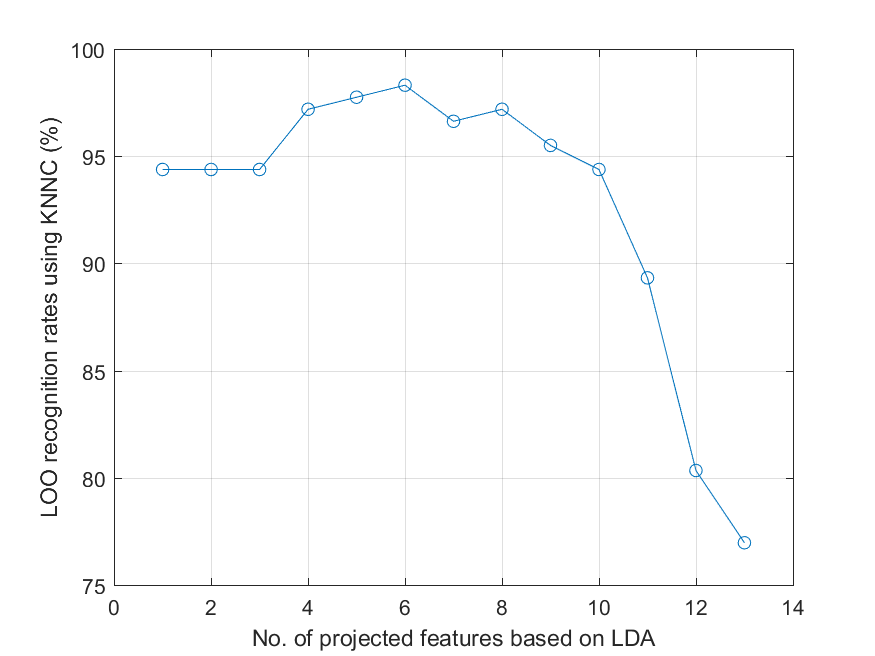The accuracy achieves its maximum of 98.31% when the dimensionality is 6. The corresponding confusion matrix is:

Example 8: ldaWineConf01.mDS=prData('wine'); DS2 = lda(DS); DS3=DS2; DS3.input=DS3.input(1:6, :); [recogRate, computedOutput] = knncLoo(DS3); confMat=confMatGet(DS3.output, computedOutput); confMatPlot(confMat);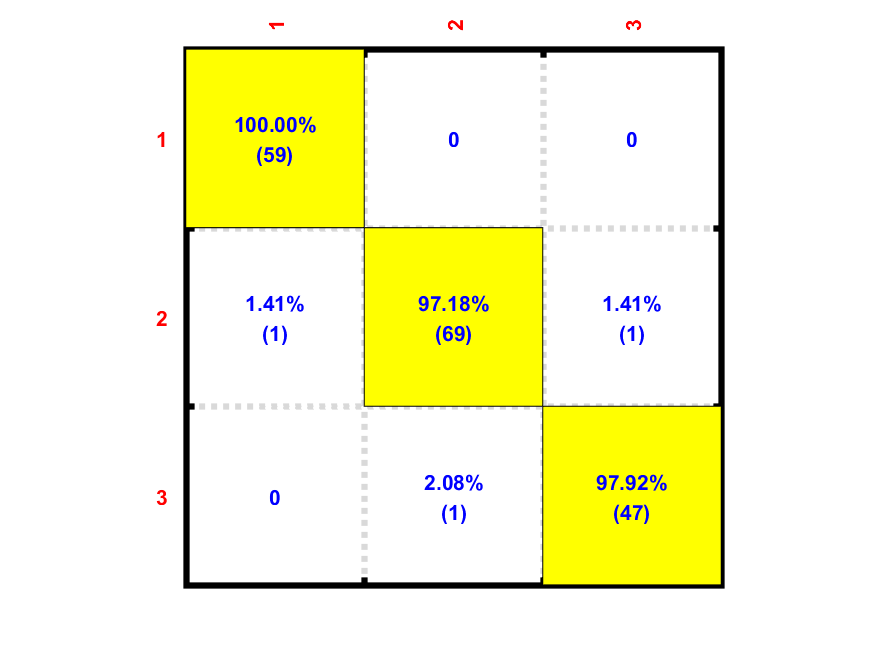If we convert the above example into a function ldaPerfViaKnncLoo.m, then we can test the effect of input (or feature) normalization:

Example 9: ldaWineDim02.mDS=prData('wine'); recogRate1=ldaPerfViaKnncLoo(DS); DS2=DS; DS2.input=inputNormalize(DS2.input); % input normalization recogRate2=ldaPerfViaKnncLoo(DS2); [featureNum, dataNum] = size(DS.input); plot(1:featureNum, 100*recogRate1, 'o-', 1:featureNum, 100*recogRate2, '^-'); grid on legend({'Raw data', 'Normalized data'}, 'location', 'northOutside', 'orientation', 'horizontal'); xlabel('No. of projected features based on LDA'); ylabel('LOO recognition rates using KNNC (%)');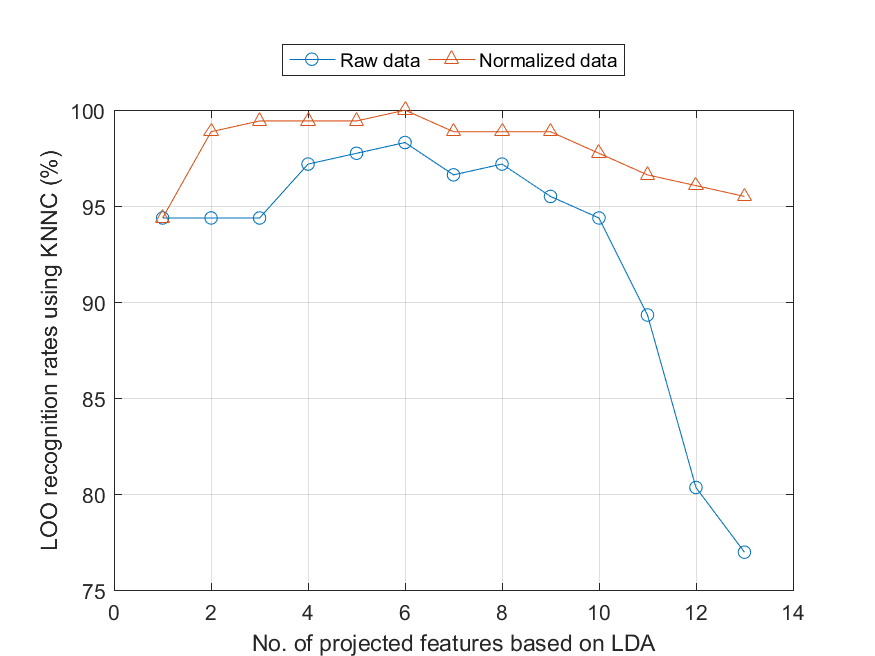For this dataset, data normalization does improve the accuracy. In particular, when the dimensionality is 6, the corresponding accuracy is 100%。

Hint
Data normalization does not always guarantee improvement in accuracy. It depends on the dataset as well as the classifier used for performance evaluation.

However, it should be aware that the above recognition rates are a little over optimistic since we used all dataset for LDA. A more objective method for LOO test should be like this:

1. Hide 1 entry and have all the other entries for LDA
2. Use the LDA basis for evaluating the hidden entry
3. Repeat the above process until each entry has served the role as the hidden data
To invoke such objective evaluation, you need to set the mode to 'exact', as follows:

Example 10: ldaWineDim03.mDS=prData('wine'); DS.input=inputNormalize(DS.input); % input normalization opt=ldaPerfViaKnncLoo('defaultOpt'); opt.mode='approximate'; tic; recogRate1=ldaPerfViaKnncLoo(DS, opt); time1=toc; opt.mode='exact'; tic; recogRate2=ldaPerfViaKnncLoo(DS, opt); time2=toc; [featureNum, dataNum] = size(DS.input); plot(1:featureNum, 100*recogRate1, 'o-', 1:featureNum, 100*recogRate2, '^-'); grid on legend({'mode=''approximate''', 'mode=''exact'''}, 'location', 'northOutside', 'orientation', 'horizontal'); xlabel('No. of projected features based on LDA'); ylabel('LOO recognition rates using KNNC (%)'); fprintf('Time for ''approximate'' mode = %g sec, time for ''exact'' mode = %g sec\n', time1, time2); Time for 'approximate' mode = 0.0543091 sec, time for 'exact' mode = 0.881161 sec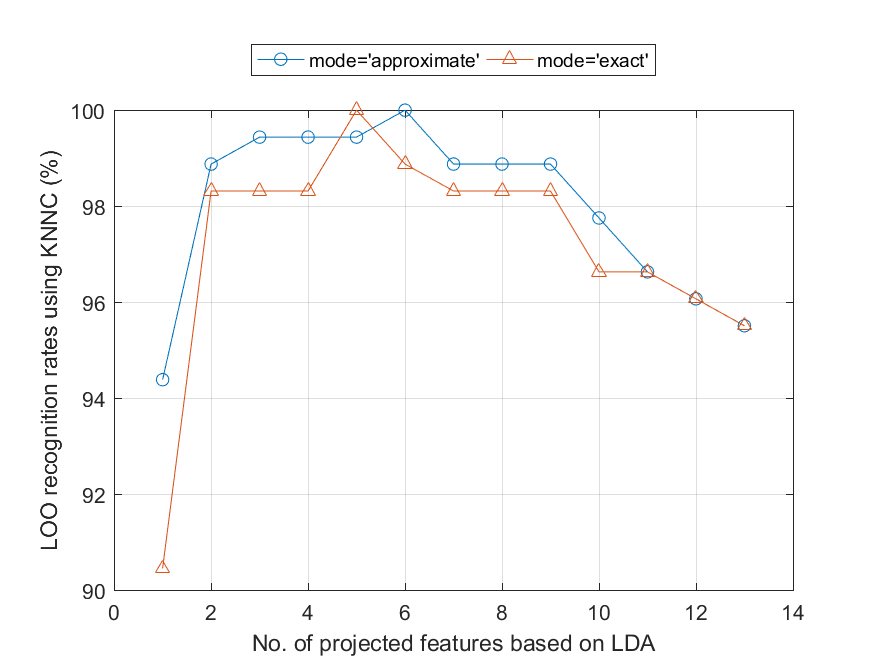Apparently, the 'exact' mode takes longer than the 'approximate' mode.

If the data dimension is larger than the data count (number of entries in the dataset), then direct use of LDA is error prone since some of the matrices are singular and the inverse operation is not reliable during LDA computation. To avoid such situation, one possible solution is to use PCA first to do dimension reduction, and then use LDA to find the best projection for classification.

References:

1. J. Duchene and S. Leclercq, "An optimal transformation for discriminant and principal component analysis", IEEE Trans. PAMI, vol. 10, pp.978-983, 1988

Appendix:

• How to prove $T=W+B$ in the crisp case: $$\begin{array}{rcl} T & = & \sum_{i=1}^n (a_i-\mu)(a_i-\mu)^T\\ & = & \sum_{i=1}^n (a_i a_i^T - \mu\mu^T)\\ & = & \sum_{i=1}^n a_i a_i^T - n \mu \mu^T\\ & = & AA^T-n\mu\mu^T\\ \end{array}$$ $$\begin{array}{rcl} W & = & \sum_{k=1}^c w_k\\ & = & \sum_{k=1}^c (A_k A_k^T - n_k \mu_k \mu_k^T)\\ & = & \sum_{k=1}^c A_k A_k^T - \sum_{k=1}^c n_k \mu_k \mu_k^T\\ & = & AA^T- \sum_{k=1}^c n_k \mu_k \mu_k^T\\ \end{array}$$ $$\begin{array}{rcl} B & = & \sum_{k=1}^c n_k (\mu_k-\mu)(\mu_k-\mu)^T\\ & = & \sum_{k=1}^c n_k \mu_k \mu_k^T - n\mu\mu^T\\ \end{array}$$ Thus we have $$T=W+B.$$
• How to prove $T=W+B$ in the fuzzy case:

Define

• $u_{k,i}$ = degree of point $i$ in class $k$.
• $\alpha_k = \sum_{i=1}^n u_{k,i}$ = size of class k.
Then $$\mu_k = \frac{\sum_{i=1}^n u_{k,i} a_i}{\sum_{i=1}^n u_{k,i}} = \frac{\sum_{i=1}^n u_{k,i} a_i}{\alpha_k} \rightarrow \alpha_k \mu_k = \sum_{i=1}^n u_{k,i}a_i.$$ Moreover, we have the following identities: $$\left\{ \begin{array}{l} \sum_{k=1}^c u_{k,i} = 1.\\ \sum_{k=1}^c \alpha_k = \sum_{i=1}^n \sum_{k=1}^c u_{k,i} = n.\\ \sum_{k=1}^c \alpha_k \mu_k = \sum_{i=1}^n (\sum_{k=1}^c u_{k,i}) a_i = \sum_{i=1}^n a_i = n \mu.\\ \end{array} \right.$$ Using the above identities, we have $$\begin{array}{rcl} T & = & \sum_{i=1}^n (a_i-\mu)(a_i-\mu)^T\\ & = & \sum_{i=1}^n (a_i a_i^T - \mu\mu^T)\\ & = & \sum_{i=1}^n a_i a_i^T - n \mu \mu^T\\ & = & AA^T-n\mu\mu^T\\ \end{array}$$ $$\begin{array}{rcl} W_k & = & \sum_{i=1}^n u_{k,i} (a_i-\mu_k)(a_i-\mu_k)^T\\ & = & \sum_{i=1}^n u_{k,i} a_i a_i^T - (\sum_{i=1}^n u_{k,i} a_i) \mu_k^T - \mu_k ({\sum_{i=1}^n u_{k,i}} a_i^T) + \sum_{i=1}^n u_{k,i}\mu_k \mu_k^T\\ & = & \sum_{i=1}^n u_{k,i} a_i a_i^T - (\alpha_k \mu_k)\mu_k^T - \mu_k (\alpha_k \mu_k^T) + \sum_{i=1}^n u_{k,i}\mu_k \mu_k^T\\ & = & \sum_{i=1}^n u_{k,i} a_i a_i^T - \alpha_k \mu_k \mu_k^T\\ \end{array}$$ $$\begin{array}{rcl} W & = & \sum_{k=1}^c w_k\\ & = & \sum_{i=1}^n \sum_{k=1}^c u_{k,i} a_i a_i^T - \sum_{k=1}^c \alpha_k \mu_k \mu_k^T\\ & = & \sum_{i=1}^n a_i a_i^T - \sum_{k=1}^c \alpha_k \mu_k \mu_k^T\\ \end{array}$$ $$\begin{array}{rcl} B & = & \sum_{k=1}^c \alpha_k (\mu_k-\mu)(\mu_k-\mu)^T\\ & = & \sum_{k=1}^c \alpha_k \mu \mu^T - (\sum_{k=1}^c \alpha_k \mu_k) \mu^T - \mu (\sum_{k=1}^c \alpha_k \mu_k)^T + (\sum_{k=1}^c \alpha_k) \mu \mu^T\\ & = & \sum_{k=1}^c \alpha_k \mu \mu^T - (n \mu) \mu^T - \mu (n \mu)^T + (n) \mu \mu^T\\ & = & \sum_{k=1}^c \alpha_k \mu_k \mu_k^T - n\mu\mu^T\\ \end{array}$$ Thus we have $T=W+B$ in the fuzzy case where each data point belong to a class to a certain degree.

Data Clustering and Pattern Recognition (資料分群與樣式辨認)